Categories

# Algorithms and Data Structures front end – the breadth and depth of the traversal of the binary tree traversal traversal

A, (FIG traversing) the depth and breadth-first priority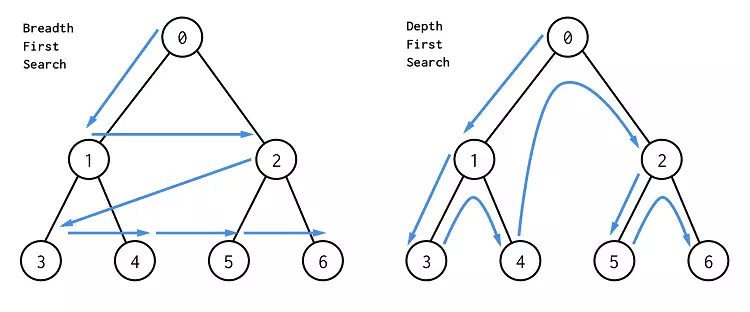– Similar binary tree preorder

The earlier point closer to the root node of the traverse.

Find the path from the starting node to the destination node, in particular, the shortest path.

First traversal BFS a vertex v from FIG sequentially access each adjacent point never visited v after visit v, then separately from the abutment point sequentially access their neighbors, and so that the “first accessed prior to the adjacent vertex dots adjacent vertex dots after access is accessed, all vertices have been visited neighbors until the figure are accessed. If at this time the figures there have not been accessed vertex, an alternative is required a vertex has not been accessed as a new starting point, repeating the process until all vertices have been drawing until accessed.

```/*Breadth traversal dom*/
//Non-recursive version

//New queues and nodes list
//Queue is not empty, remove the first element, into the Push Nodes, adds the children into the cycle queue
//Return nodes
let nodes = []
let queue = []
if(node){
queue.push(node)
while(queue.length){
let item = queue.shift()
let children = item.children
nodes.push(item)
//Queues, FIFO
// nodes = [] queue= [parent]
// nodes = [parent] queue= [child1,child2,child3]
// nodes = [parent, child1] queue= [child2,child3,child1-1,child1-2]
for(let i = 0;i){
queue.push(children[i])
}
}
}
return nodes
}```

Depth-first search (DFS) stack implementation

– a similar level of binary tree traversal

Earlier access node may not be closer to the root node.

Thus, the first path you find in DFS may not be the shortest path.

The initial state is assumed that the figures were not all vertices is accessed from a vertex v, firstly access the vertex followed from its various neighbors have not been accessed depth-first search graph traversal, until all the figures and v there are similarities path to vertices have been visited. If this fashion not have access to the other vertex, the vertex alternatively as a starting point has not been accessed, repeating the process until all vertices have been drawing until accessed.

```/*Depth traversing dom*/
//Non-recursive version
let deepTraversal = (node) => {
let stack = []
let nodes = []
if (node) {
//Push currently processed node
stack.push(node)
while (stack.length) {
let item = stack.pop()
let children = item.children
nodes.push(item)
// node = [] stack = [parent]
// node = [parent] stack = [child3,child2,child1]
// node = [parent, child1] stack = [child3,child2,child1-2,child1-1]
// node = [parent, child1-1] stack = [child3,child2,child1-2]
for (let i = children.length - 1; i >= 0; i--) {
stack.push(children[i])
}
}
}
return nodes
}
//Recursive version
let deepTraversal1 = (node) => {
let nodes =[]
if(node!== null) {
nodes.push(node)
let children = node.children;
for(let i = 0;i){
nodes = nodes.concat(deepTraversal(children[i]))
}
}
return nodes;
}
let parent = document.querySelector('.parent');
let joe = deepTraversal(parent);
console.log(joe);```

Application: search for a path node, add path property (BFS the shift (queue), using the DFS pop (Stack))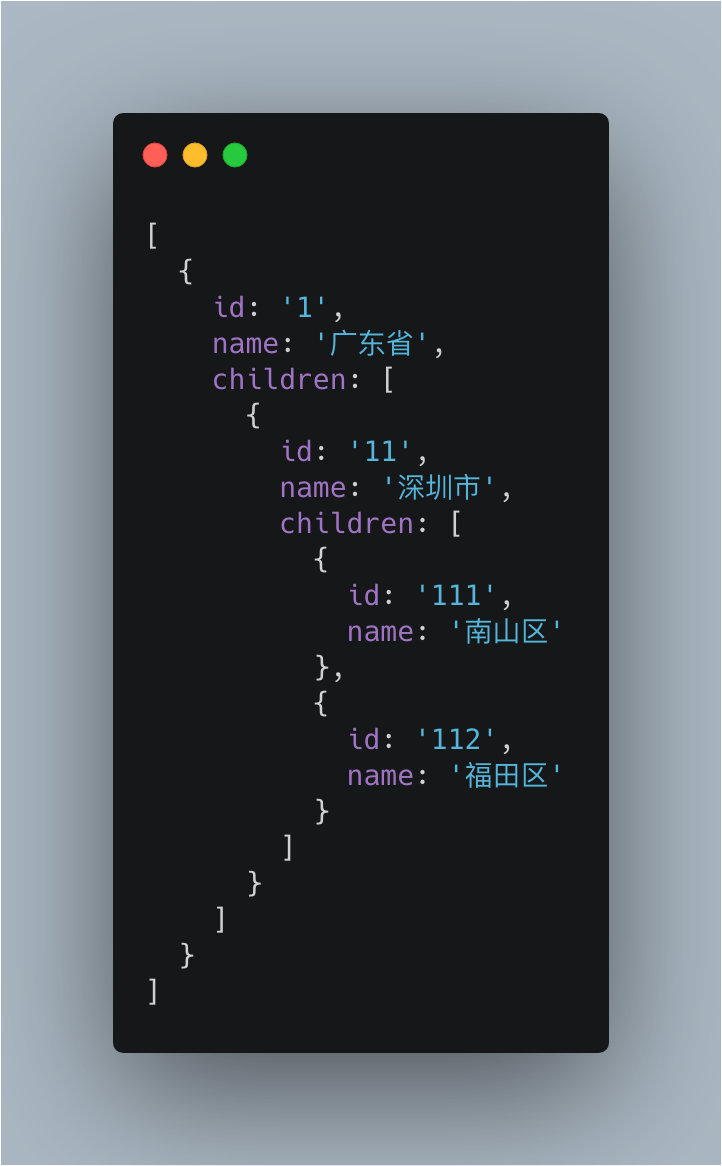· Bfs implemented using a queue, the cycle is done push => shift => push => shift

· Dfs use stack implementation cycle to do is push => pop => push => pop

```/*To find the corresponding name breadth traversal path queue implementation bfs*/
function bfs(target, name) {
const queue = [...target]
do {
const current = queue.shift()
if (current.children) {
queue.push(...current.children.map(x => ({ ...x, path: (current.path || current.name) + '-' + x.name })))
}
if (current.name === name) {
return current//Returns the entire audience
}
} while(queue.length)
return undefined
} ```
```/*Find the path name corresponding to the depth of traversal stack implementation dfs*/
function dfs(target,name){
const stack = [...target]
do{
const current = stack.pop()
if(current.children){
stack.push(...current.children.map(x => ({...x,path:(current.path || current.name) + '-' + x.name})))
}
if(current.name === name){
return current.path//Return path attribute the entire target object
}

}while(stack.length)
return undefined
}```
```/*Public search method, mode default bfs*/
function commonSearch(target, name, mode) {
const stackOrQueue = [...target]
do {
const current = stackOrQueue[mode === 'dfs' ? 'pop' : 'shift']()
if (current.children) {
stackOrQueue.push(...current.children.map(x => ({ ...x, path: (current.path || current.name) + '-' + x.name })))
}
if (current.name === name) {
return current
}
} while(stackOrQueue.length)
return undefined
}```

```/*Search test area*/
const data = [{
id: '1',
name: '广东省',
children: [
{
id: '12',
name: '广州市',
children: [
{
id:'121',
name: '天河区'
},
{
id:'122',
name: '荔湾区'
}
]
}
]
}]
console.log(dfs(data,'天河区'))```

· Result 1: Returns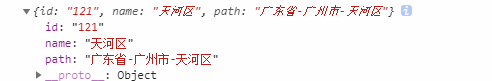· Results: Returns the path attribute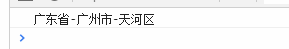Second, the (binary tree traversal) subsequent the preamble sequence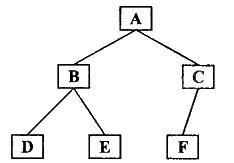Focus of the focus, the best control of both recursive and non-recursive version.

Recursive version is easy to write, but really investigate non-recursive version of the basic skills.

· Preorder traversal of a binary tree (the root node N before the left and right subtrees) NLR – ABDECF

· Binary tree traversal sequence (N around the root subtree) LNR – DBEAFC

· Postorder binary tree (root node N in the left and right subtrees) LRN – DEBFCA

```/*Recursive wording*/
/*prologue*/
const preorderTraversal = function (root, array=[]) {
if(root) {
array.push(root.val);
preorderTraversal(root.left, array);
preorderTraversal(root.right, array);
}
return array;
}
/*In order*/
const inorderTraversal = function(root, array= []) {
if(root) {
inorderTraversal(root.left, array);
array.push(root.val);
inorderTraversal(root.right, array);
}
return array;
}
/*After the order*/
const postorderTraversal = function(root, array = []) {
if(root){
postorderTraversal(root.left, array);
postorderTraversal(root.right, array);
array.push(root.val);
}
return array;
}

/*Non-recursive wording*/
/*prologue*/
const preorderTraversal1 = function (root) {
const result = [];
const stack = [];
let current = root;
while(current||stack.length > 0){
while(current){
//Take root value, the left node stack, recycled to the left node is empty
result.push(current.val);
stack.push(current);
current = current.left;
}
//A stack node, and then traverse right node
current = stack.pop();
current = current.right;
}
return result;
}
/*In order*/
const inorderTraversal1 = function (root) {
const result = [];
const stack = [];
let current = root;
while(current||stack.length > 0){
while(current){
//Left node onto the stack, loop to the left node is empty
stack.push(current);
current = current.left;
}
//A stack node, the access node values, and then traverse right node
current = stack.pop();
result.push(current.val);
current = current.right;
}
return result;
}
/*After the order*/
const postorderTraversal1 = function (root) {
const result = [];
const stack = [];
let last = null;
let current = root;
while(current || stack.length > 0) {
while(current) {
//Left node onto the stack, loop to the left node is empty
stack.push(current);
current = current.left;
}
//Take the last node within the stack is empty or has been determined is traversed, then the node values ​​passed
current = stack[stack.length-1];
if(!current.right || current.right == last){
//Stack node
current = stack.pop();
result.push(current.val);
last = current;
current = null;
//Continue to cycle popped
} else {
//Taking the right node traversal operation done
current = current.right
}
}
return result;
}
/*Test Case*/
const data = {
val:1,
left:{
val:2,
left:{
val:4,
},
right:{
val:5
}
},
right:{
val:3,
left:{
val:6
},
right:{
val:7
}
}
}
console.log('递归计算结果')
console.log(preorderTraversal(data))
console.log(inorderTraversal(data))
console.log(postorderTraversal(data))
console.log('非递归计算结果')
console.log(preorderTraversal1(data))
console.log(inorderTraversal1(data))
console.log(postorderTraversal1(data))

//Recursive calculations
[ 1, 2, 4, 5, 3, 6, 7 ]
[ 4, 2, 5, 1, 6, 3, 7 ]
[ 4, 5, 2, 6, 7, 3, 1 ]
//Non-recursive calculations
[ 1, 2, 4, 5, 3, 6, 7 ]
[ 4, 2, 5, 1, 6, 3, 7 ]
[ 4, 5, 2, 6, 7, 3, 1 ]```